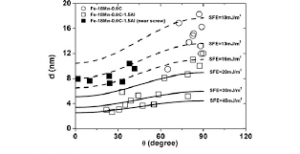## How to Calculate and Solve for Partial Dislocation Separation | Fracture MechanicsThe image above represents partial dislocation separation.

To compute for partial dislocation separation, four essential parameters are needed and these parameters are Shear Modulus (G), Burger Vector (b2), Burger Vector (b3and Stacking Fault Energy (γ).

The formula for calculating partial dislocation separation:

d = Gb2b3 / 2πγ

Where:

d = Partial Dislocation Separation
G = Shear Modulus
b2 and b3 = Burger Vectors
γ = Stacking Fault Energy

Let’s solve an example;
Find the partial dislocation separation when the shear modulus is 4. the burger vectors is 8 and 10, the stacking fault energy is 14.

This implies that;

G = Shear Modulus = 4
b2 and b3 = Burger Vectors = 8 and 10
γ = Stacking Fault Energy = 14

d = Gb2b3 / 2πγ
d = (4)(8)(10) / 2π(14)
d = 320 / 87.96
d = 3.64

Therefore, the partial dislocation separation is 3.64 m.